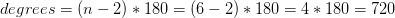## Example Questions

200

170

210

180

190

### Example Question #2 : How To Find An Angle In A Hexagon

If a triangle has 180 degrees, what is the sum of the interior angles of a regular octagon?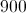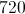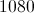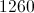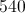Explanation:

The sum of the interior angles of a polygon is given by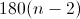where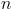= number of sides of the polygon.  An octagon has 8 sides, so the formula becomes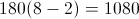### Example Question #22 : Sat Mathematics

Find the sum of all the inner angles in a hexagon.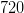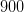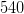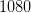Explanation:

To solve, simply use the formula to find the total degrees inside a polygon, where n is the number of vertices.

In this particular case, a hexagon means a shape with six sides and thus six vertices.

Thus,